Theory and Modern Applications

# Existence of mild solutions for impulsive neutral Hilfer fractional evolution equations

## Abstract

In this paper, we investigate the existence of mild solutions for neutral Hilfer fractional evolution equations with noninstantaneous impulsive conditions in a Banach space. We obtain the existence results by applying the theory of resolvent operator functions, Hausdorff measure of noncompactness, and Sadovskii’s fixed point theorem. We also present an example to show the validity of obtained results.

## Introduction

Fractional calculus primarily involves the description of fractional-order derivatives and integral operators . In the last few decades, it has gained significant importance because of its wide range of applicability in diverse scientific domains. Fractional differential equations (FDEs) are among the strongest tools of mathematical modeling and are successfully employed to model complex physical and biological phenomena like anomalous diffusion, viscoelastic behavior, power laws, and automatic remote control systems. In the available literature, notable definitions of fractional derivatives were given by famous mathematicians, but the most commonly used are the Riemann–Liouville (RL) and Caputo derivatives [1, 2, 21, 22, 24, 26, 29, 39]. Thus FDEs involving the RL fractional derivative or Caputo derivative have considered frequently for investigating the existence of mild solutions. However, little attention has been devoted to FDEs with generalized fractional derivatives. The Hilfer fractional derivative (HFD), a generalization of the RL fractional derivative was first introduced by Hilfer [4, 11, 15, 16]. The existence and uniqueness of general initial and boundary value problems involving HFD were first examined by Furati and Kassim  and Wang and Zhang , respectively. Thereafter, by means of the noncompact measure method Gu and Trujilo  defined mild solutions for FDEs involving HFD. Later on, Gou and Li  proved the existence of mild solutions for Sobolev-type Hilfer fractional evolution equations with boundary conditions. In [17, 32, 33] the authors considered Hilfer FDEs with nonlocal conditions for investigating approximate controllability and existence of solutions. Recently, Subashini et al.  obtained mild solutions for Hilfer integro-differential equations of fractional order by means of Monch’s fixed point technique and noncompact measure. FDEs involving HFD are widely applicable in biomedical research. These equations are successfully employed to model the irregular boundaries of biological cells and microscopic fluctuations of biomedical matters .

Hernandez et al.  first introduced the concept of noninstantaneous impulsive conditions. These conditions appeared in the mathematical description of real-world dynamical processes experiencing a sudden change over a short interval of time like DNA sequences, heart beat intervals, optimal control models, and so on . In the published work, FDEs involving either RL or Caputo derivative are commonly considered with impulsive conditions for obtaining mild solutions [5, 7, 20, 27, 30]. However, Sousa et al.  for the first time obtained the mild solutions for Hilfer FDEs with noninstantaneous impulsive conditions. Similalrly, no existence results have been established for neutral Hilfer FDEs in contrast to neutral FDEs with RL or Caputo derivative [9, 25, 35, 40]. Such equations have a bundle of applications in physics, biology, and electrical engineering. Thus, to make a little contribution to existing works, we consider the neutral Hilfer FDEs with impulsive conditions of the mentioned form for obtaining mild solutions. We obtain an existence result with the help of fixed point theory, which is proven to be an authoritative modeling tool for obtaining exact or approximate solutions of FDEs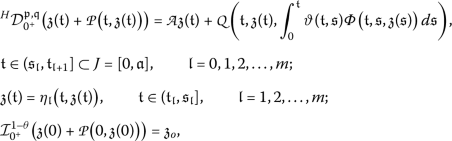(1.1)

where $${\mathcal{I}}_{0^{+}}^{1-\theta }$$ is the RL fractional integral, $${}^{H}{\mathcal{D}}^{\mathfrak{p}, \mathfrak{q}}_{0^{+}}$$ is the HFD of order $$(\mathfrak{p},\mathfrak{q})$$ with $$0\le \mathfrak{p}<1$$, $$0\le \mathfrak{q}\le 1$$, and $$0 \le \theta =\mathfrak{p} +\mathfrak{q}- \mathfrak{p}\mathfrak{q}\le 1$$, the linear operatoris the infinitesimal generator of a strongly continuous semigroupin a Banach space, $$0=\mathfrak{t}_{o}=\mathfrak{s}_{o}<\mathfrak{t}_{1}<\mathfrak{s}_{1}< \mathfrak{t}_{2}<\cdots<\mathfrak{t}_{\mathfrak{l}}<\mathfrak{s}_{\mathfrak{l}}<\mathfrak{t}_{\mathfrak{l}+1}=\mathfrak{a}$$ is a partition of $$[0, \mathfrak{a}]$$, $$\mathfrak{a}>0$$, $$\mathfrak{l}=1, 2, \ldots, m$$,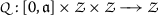andare appropriate functions satisfying some assumptions to be discussed later, and a continuous function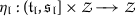characterizes the impulsive conditions and. The properties of functions $$\vartheta :\Delta \longrightarrow \mathbb{R}$$ and, $$\Delta =(\mathfrak{t}, \mathfrak{s}) \in [0, \mathfrak{a}]\times [0, \mathfrak{a}]$$ are specified in later section.

This manuscript is structured as follows. In Sect. 2, we discuss the Hilfer fractional derivative, Hausdorff measure of noncompactness, and mild solutions of equation (1.1) along with some basic results and lemmas. In later section, we obtain existence results by means of fixed point technique, measure of noncompactness, and Lebesgue dominated convergence theorem. We confirm the validity of obtained results by offering an example in the last section.

## Preliminaries

Letdenote the complete normed linear space of continuous functions $$\mathfrak{z}(\mathfrak{t})$$ defined on the interval $$\mathcal {J}=[0, \mathfrak{a}]$$ with $$\|\mathfrak{z}\|=\sup{\mathfrak{t}\in \mathcal {J}}\| \mathfrak{z}(\mathfrak{t})\|$$. We define the Banach space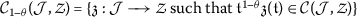with norm $$\|\mathfrak{z}\|_{\mathcal{C}_{1-\theta }}=  \sup_{0\le \mathfrak{t}\le \mathfrak{a}}|\mathfrak{t}^{1- \theta }\mathfrak{z}(\mathfrak{t})|$$.

We will establish the existence results in the Banach spacefor $$\mathfrak{l}=1,2, \ldots,m$$ with the corresponding norm

$$\Vert {\mathfrak{z}} \Vert _{\mathcal{PC}_{1-\theta }}=\max \Bigl\{ \sup _{\mathfrak{t}\in \mathcal {J}}\|{\mathfrak{t}}^{1- \theta }\mathfrak{z}\bigl({ \mathfrak{t}}^{+}\bigr), \sup_{{\mathfrak{t}}\in \mathcal {J}} \bigl\Vert { \mathfrak{t}}^{1- \theta }\mathfrak{z}\bigl({\mathfrak{t}}^{-}\bigr) \bigr\Vert \Bigr\} .$$

Bywe denotes the family of bounded linear operators defined on, and by $$\{{\mathcal{W}}_{\mathfrak{p}, \mathfrak{q}}({\mathfrak{t}}) \}_{{ \mathfrak{t}}\ge 0}$$ the $$(\mathfrak{p} -\mathfrak{q})$$-resolvent operator or the $$\mathfrak{q}$$-times integrated $$\mathfrak{p}$$-resolvent operator generated by.

### Definition 2.1

()

The Hilfer fractional derivative of order $$n-1 \le \mathfrak{p} < n$$, $$n\in \mathbb{N}$$; $$0\le \mathfrak{q} \le 1$$, with lower limit $$\mathfrak{c}$$ is defined as

$${\mathcal{D}}^{\mathfrak{p}, \mathfrak{q}}_{\mathfrak{c}^{+}}{ f}( \mathfrak {t})= { \mathcal{I}}^{\mathfrak{p} (n-\mathfrak{q})}_{ \mathfrak{c}^{+}}\frac{d}{dt}{\mathcal{I}}^{(1-\mathfrak{p}) (n- \mathfrak{q})}_{\mathfrak{c}^{+}}{ f}(t)={\mathcal{I}}^{\mathfrak{p} (n- \mathfrak{q})}_{\mathfrak{c}^{+}}{\mathcal{D}}^{\mathfrak{q}+\mathfrak{p} n- \mathfrak{q} \mathfrak{p}}_{\mathfrak{c}^{+}}{f}(t),$$

where $${\mathcal{I}}^{\mathfrak{p} (n-\mathfrak{q})}_{\mathfrak{c}^{+}}$$ is the RL integral, and $${\mathcal{D}}^{\mathfrak{q}+\mathfrak{p} n-\mathfrak{p} \mathfrak{q}}_{ \mathfrak{a}^{+}}$$ is the RL derivative.

### Lemma 2.1

()

Let$$0<\mathfrak{p}<1$$, $$0\leq \mathfrak{q}\leq 1$$, and$$\theta =\mathfrak{p}+\mathfrak{q}-\mathfrak{p}\mathfrak{q}$$. If$$f \in {\mathcal{C}}_{1-\theta }[\mathfrak{c}, \mathfrak{d}]$$is such that$${\mathcal{D}}_{\mathfrak{c}^{+}}^{\theta }f\in {\mathcal{C}}_{1-\theta }[ \mathfrak{c}, \mathfrak{d}]$$, then

$${\mathcal{I}}_{\mathfrak{c}^{+}}^{\theta }{\mathcal{D}}_{\mathfrak{c}^{+}}^{ \theta }f={ \mathcal{I}}_{\mathfrak{c}^{+}}^{\mathfrak{p}}{\mathcal{D}}^{ \mathfrak{p}, \mathfrak{q}}_{\mathfrak{c}^{+}}f \quad \textit{and}\quad {\mathcal{D}}_{ \mathfrak{c}^{+}}^{\theta }{\mathcal{I}}_{\mathfrak{c}^{+}}^{\theta }f= { \mathcal{D}}_{\mathfrak{c}^{+}}^{\mathfrak{q}(1-\mathfrak{p})}f.$$

### Lemma 2.2

()

Let$$0<\mathfrak{p}<1$$and$$0\le \theta \leq 1$$. If$$f\in {\mathcal{C}}_{1-\theta }[\mathfrak{c}, \mathfrak{d}]$$and$${\mathcal{I}}_{\mathfrak{c}^{+}}^{1-\mathfrak{p}}f\in {\mathcal{C}}_{ \theta }^{1}[\mathfrak{c}, \mathfrak{d}]$$, then

$${\mathcal{I}}_{\mathfrak{c}^{+}}^{\mathfrak{p}}{\mathcal{D}}_{\mathfrak{c}^{+}}^{ \mathfrak{p}}f(x)=f(x)- \frac{{\mathcal{I}}^{1-\mathfrak{p}}_{\mathfrak{c}^{+}}f(\mathfrak{c})}{\varGamma (\mathfrak{p})}(x- \mathfrak{c})^{\mathfrak{p}-1}, \quad \forall x\in ( \mathfrak{c}, \mathfrak{d}].$$

### Definition 2.2

()

The Hausdorff measure of noncompactness on a bounded subsetof Banach spaceis the mapping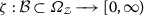defined as

$$\zeta ({\mathcal{B}})=\inf \biggl\{ \epsilon >0: {\mathcal{B}}= \bigcup { \mathcal{B}}_{i} \text{ with radii of } { \mathcal{B}}_{i} \le \epsilon \text{ for } i=1, 2, \ldots, m \biggr\} .$$

### Lemma 2.3

([3, 23])

The measure of noncompactnessζdefined on bounded subsets$$\mathcal{ P}$$and$$\mathcal{ Q }$$of a Banach spacehas following properties:

1. 1

$$\zeta (\mathcal{P}) = 0$$iff$${ \mathcal{P}}$$is a relatively compact set.

2. 2

$$\mathcal{\mathcal{P}} \subset \mathcal{Q} \implies \zeta (\mathcal{P}) \le \zeta (\mathcal{Q})$$.

3. 3

$$\zeta (\bar{\mathcal{P}})=\zeta (\mathcal{P})$$.

4. 4

$$\zeta (\mathcal{P}\cup \mathcal{Q})= \max \lbrace \zeta ( \mathcal{P}), \zeta (\mathcal{Q}) \rbrace$$.

5. 5

$$\zeta (\mathfrak{a}\mathcal{P})= |\mathfrak{a}|\zeta (\mathcal{P})$$, $$\forall \mathfrak{a} \in \mathbb{R}$$.

### Lemma 2.4

([3, 23])

For a bounded set, there is a denumerable set$${\mathcal{D}}_{o} \subset {\mathcal{D}}$$such that$$\zeta ({\mathcal{D}}_{o})\le \zeta ({\mathcal{D}})$$.

### Lemma 2.5

()

For a bounded and equicontinuous function, the Hausdorff measure of noncompactness$$\zeta (\mathcal{G}({\mathfrak{t}}) )$$is continuous on$$\mathcal {J}$$, and$$\zeta (\mathcal{G})= \max_{{\mathfrak{t}}\in \mathcal {J}} \zeta ( \mathcal{G}({\mathfrak{t}}) )$$.

### Lemma 2.6

()

Let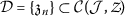be a bounded denumerable subset of. Then$$\zeta (\mathcal{D}({\mathfrak{t}}) )$$is Lebesgue integrable on, and

$$\zeta \biggl( \int \mathfrak{z}_{n}({\mathfrak{t}})\,d{\mathfrak{t}}: n \in \mathbb{N} \biggr)\le \int \zeta \bigl( \mathfrak{z}_{n}({\mathfrak{t}}) \bigr) \,d{ \mathfrak{t}}.$$

### Lemma 2.7

()

After applying Lemmas2.1and2.2, the system of fractional nonlinear differential equations (1.1) reduces to the following integral equation: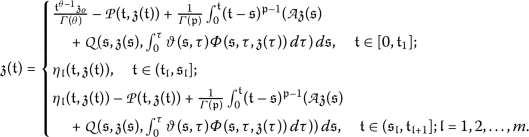(2.1)

### Definition 2.3

([13, 31])

A mild solution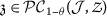of problem (1.1) is the solution of the corresponding its integral form (2.1)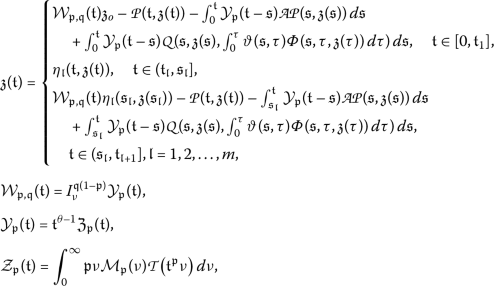(2.2)

where $$\mathcal{M}_{\mathfrak{p}}(\nu )$$ is the Wright function defined as

$$\mathcal{M}_{\mathfrak{p}}(\nu )=\sum_{n=1}^{\infty } \frac{(-\nu )^{n-1}}{(n-1)\varGamma (1-\mu n)},\quad 0< \mu < 1,\nu \in \mathbb{C},$$

and satisfying the equality

$$\int _{0}^{\infty } \nu ^{\sigma } \mathcal{M}_{\mathfrak{p}}(\nu )\,d\nu = \frac{\varGamma (1+\sigma )}{\varGamma (1+\mathfrak{p}\sigma )},\quad \nu , \sigma \ge 0.$$

This definition of a mild solution is obtained by means of the Laplace transform of the Hilfer fractional derivative.

### Remark 2.1

The Laplace transform of the Hilfer derivative of a function $${f(\mathfrak{t})}$$ of order $$0<\mathfrak{p}<1$$ and $$0<\mathfrak{q}<1$$ is as follows :

$$L \bigl\lbrace {\mathcal{D}}^{\mathfrak{p}, \mathfrak{q}}_{a^{+}}f( \mathfrak{t});{ \mathfrak{s}} \bigr\rbrace = {\mathfrak{s}}^{\mathfrak{p}} f(\mathfrak{s})- { \mathfrak{s}}^{\mathfrak{p} (\mathfrak{q} -1)}{ \mathcal{I}}_{0+}^{(1-\mathfrak{p})(1-\mathfrak{q})}f(0+),$$

where $${\mathcal{I}}_{0+}^{(1-\mathfrak{p})(1-\mathfrak{q})}f(0+)$$ is the RL fractional integral of order $$(1-\mathfrak{p})(1-\mathfrak{q})$$.

### Remark 2.2

([6, 13])

Let us assume

1. 1

The strong continuity of linear operators $$\lbrace {\mathcal{W}}_{\mathfrak{p}, \mathfrak{q}}(\mathfrak{t}) \rbrace _{\mathfrak{t}>0}$$ and $$\lbrace {\mathcal{Y}}_{\mathfrak{p}}(\mathfrak{t}) \rbrace _{ \mathfrak{t}>0}$$ with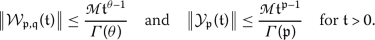2. 2

The norm continuity of familyfor $$\mathfrak{t}>0$$.

## Existence result

In the beginning of this section, we introduce some assumptions required to obtain the desired result:

(H1)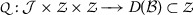is a Carathéodory function, that is,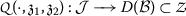is measurable for all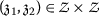,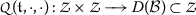is continuous a.e. for $$\mathfrak{t}\in \mathcal {J}$$, and there exist $$\psi _{1}\in L_{\frac{1}{\mathsf{r}}}( \mathcal {J}, \mathbb{R}^{+})$$, $$\frac{1}{\mathsf{r}}>1$$, and a continuous function $$\psi _{2}$$ such that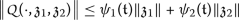for almost all $$\mathfrak{t}\in \mathcal {J}$$.

(H2) There exist functions $${\hat{\phi }}_{1}$$, $${\hat{\phi }}_{2}\in L_{\frac{1}{\mathsf{r}}}( \mathcal {J}, \mathbb{R}^{+})$$ and constantssuch that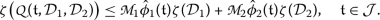for any bounded, equicontinuous, and countable sets, $${\mathfrak{l}}=1, 2$$.

(H3)is bounded and Lipschitz continuous, that is, there existand $\mathcal{LP}\in \left(0,1\right)$ such that(H4) The impulsive function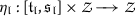is Lipschitz continuous, that is, there exist $$\mathcal{K}_{\eta _{\mathfrak{l}}}>0$$, $$\mathfrak{l}=1, 2, \ldots, m$$, such that for all,

$$\bigl\Vert \eta _{\mathfrak{l}}(\mathfrak{t}, \mathfrak{z}_{1})- \eta _{\mathfrak{l}} ( \mathfrak{t}, \mathfrak{z}_{2}) \bigr\Vert \le \mathcal{K}_{\eta _{\mathfrak{l}}} \Vert \mathfrak{z}_{1}- \mathfrak{z}_{2} \Vert .$$

(H5)is a Carathéodary function, and there exist $$\mathfrak{\bar{m}}:\Delta \longrightarrow \mathbb{R}$$ with(H6) For a bounded setand $$0\le \mathfrak{s}\le \mathfrak{t}\le \mathfrak{a}$$, there exists a function $$\xi : \Delta \longrightarrow \mathbb{R}$$ such that

$$\zeta \bigl( \varPhi (\mathfrak{t}, \mathfrak{s}, \mathcal{D}_{1}) \bigr) \le \xi (\mathfrak{t}, \mathfrak{s})\zeta (\mathcal{D}_{1}),$$

where

$$\xi ^{*}= \sup \int _{0}^{\mathfrak{t}}\xi (\mathfrak{t}, \mathfrak{s})\,d \mathfrak{s}< \infty .$$

(H7) $$\vartheta ^{*}=\sup \lbrace \vartheta (\mathfrak{t}, \mathfrak{s}): 0\le \mathfrak{s} \le \mathfrak{t} \rbrace$$ is bounded and measurable on $$\mathcal {J}$$ along with the continuity of the function $$\vartheta _{\mathfrak{t}}: \mathcal {J}\longrightarrow L^{\infty }( \mathcal {J}, \mathbb{R})$$ defined as $$\vartheta _{t}(s)=\vartheta (t, s)$$.

### Theorem 3.1

The equation system (1.1) has at least one mild solution in the space$${\mathcal{PC}}_{1-\theta }$$if assumptions (H1)–(H7) hold along with the following conditions: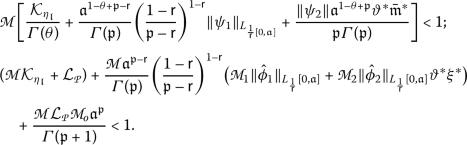### Proof

To prove the existence of a mild solution for equation system (1.1), it is sufficient to prove the existence of a solution the corresponding integral form (2.2).

Define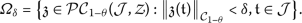Clearly, $$\varOmega _{\delta }$$ is a closed convex bounded subset of. Define the operator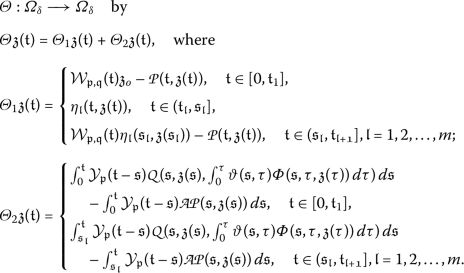The operator Θ is well defined.

We establish our results in six steps.

Step 1. We show that $${\varTheta }\mathfrak{z} \in \mathcal{P\mathcal{C}}_{1-\theta }$$ for $$\mathfrak{z} \in {\mathcal{P}\mathcal{C}}_{1-\theta }$$, that is, $${\mathfrak{t}}^{1-\theta }{\varTheta }\mathfrak{z}(\mathfrak{t})$$ is a continuous function for $$\mathfrak{t}\in (\mathfrak{t_{l}}, \mathfrak{t_{l+1}}]$$, $$\mathfrak{l}=0,1,2,\ldots,m$$. For $$0\le \tilde{\mathfrak{t}}\le \mathfrak{t} <\mathfrak{t}_{1}$$, we consider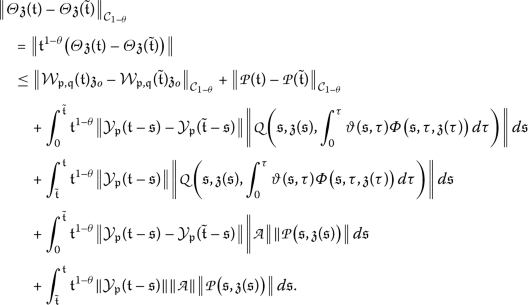Substituting $$\tilde{\mathfrak{t}}-\mathfrak{s}=\mathfrak{s}_{1}$$ into the third and fifth terms, we have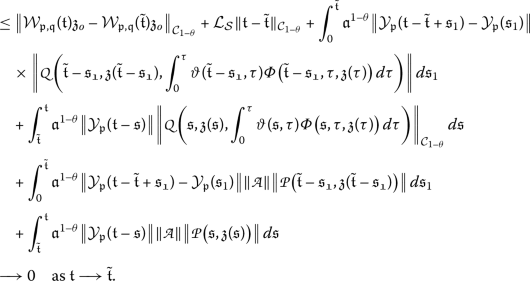This proves the continuity of $$\mathfrak{t}^{1-\theta } { \varTheta }\mathfrak{z}(\mathfrak{t})$$ for $$\mathfrak{t}\in [0, \mathfrak{t}_{1}]$$, that is,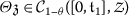.

Using the continuity of noninstantaneous impulsive functions $$\eta _{\mathfrak{l}} (\mathfrak{t}, \mathfrak{z}(\mathfrak{t}) )$$ for $$\mathfrak{l}=1,2,\ldots,m$$ and applying the similar procedure as before, we easily see that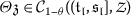and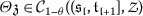. Thus we conclude that $${\varTheta }\mathfrak{z}\in {\mathcal{P}\mathcal{C}}_{1-\theta }$$ for $$\mathfrak{z} \in {\mathcal{P}\mathcal{C}}_{1-\theta }$$.

Step 2. We show that Θ maps the bounded closed convex set $${\varOmega _{\delta }}$$ onto itself, that is, $${\varTheta }\mathfrak{z}\in \varOmega _{\delta }$$ for $$\mathfrak{z} \in \varOmega _{\delta }$$. Suppose the contrary, that is, there are $$\mathfrak{z}\in \varOmega _{\delta }$$ and $$\mathfrak{t} \in \mathcal {J}$$ such that $$\|{\varTheta }\mathfrak{z}\|_{\mathfrak{C}_{1-\theta }}> \delta$$. Let us evaluate $$\|{\varTheta }\mathfrak{z}\|$$ for $$\mathfrak{t}\in [0, \mathfrak{t}_{1}]$$, $$(\mathfrak{t}_{\mathfrak{l}}, \mathfrak{s}_{\mathfrak{l}}]$$, and $$(\mathfrak{s}_{\mathfrak{l}}, \mathfrak{t}_{\mathfrak{l}+1}]$$, $$\mathfrak{l}=1, 2, \ldots, m$$.

For $$\mathfrak{t}\in [0, \mathfrak{t}_{1}]$$, we have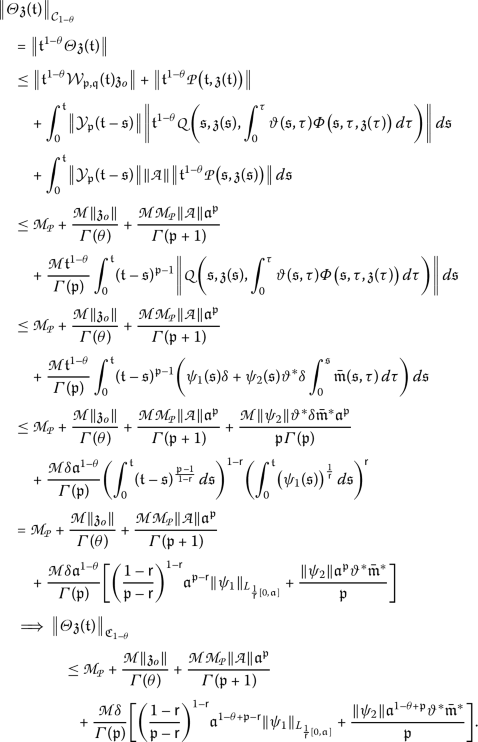(3.1)

For $$\mathfrak{t}\in (\mathfrak{t}_{\mathfrak{l}}, \mathfrak{s}_{\mathfrak{l}}]$$, $${\mathfrak{l}}=1, 2, \ldots, m$$, we have

\begin{aligned}& \begin{aligned}[b] \bigl\Vert {\varTheta }\mathfrak{z}( \mathfrak{t}) \bigr\Vert _{\mathcal{C}_{1-\theta }}&= \bigl\Vert \mathfrak{t}^{1-\theta }{ \varTheta }\mathfrak{z}(\mathfrak{t}) \bigr\Vert = \bigl\Vert \mathfrak{t}^{1-\theta }\eta _{\mathfrak{l}} \bigl( \mathfrak{t}, \mathfrak{z}( \mathfrak{t}) \bigr) \bigr\Vert = \bigl\Vert \eta _{\mathfrak{l}} \bigl( \mathfrak{t}, \mathfrak{z}(\mathfrak{t}) \bigr) \bigr\Vert _{\mathcal{C}_{1-\theta }} \\ & = \bigl\Vert \eta _{\mathfrak{l}} \bigl( \mathfrak{t}, \mathfrak{z}( \mathfrak{t}) \bigr)- \eta _{\mathfrak{l}}(t,0)+\eta _{\mathfrak{l}}( \mathfrak{t},0) \bigr\Vert _{ \mathfrak{C}_{1-\theta }} \\ & \le \mathcal{K}_{\eta _{\mathfrak{l}}} \bigl\Vert \mathfrak{z}(\mathfrak{t}) \bigr\Vert _{ \mathcal{C}_{1-\theta }}+ \bigl\Vert \eta _{\mathfrak{l}}(\mathfrak{t}, 0) \bigr\Vert _{ \mathcal{C}_{1-\theta }} \\ & \le \mathcal{K}_{\eta _{\mathfrak{l}}}\delta + {N},\quad \text{where } N= \sup \bigl\Vert {\eta _{\mathfrak{l}}}(\mathfrak{t}, 0) \bigr\Vert _{\mathcal{C}_{1-\theta }} \end{aligned} \\& \quad \implies \bigl\Vert {\varTheta }\mathfrak{z}(\mathfrak{t}) \bigr\Vert _{\mathcal{C}_{1- \theta }} \le \mathcal{K}_{\eta _{\mathfrak{l}}} \delta + N. \end{aligned}
(3.2)

For $$\mathfrak{t}\in (\mathfrak{s}_{\mathfrak{l}}, \mathfrak{t}_{\mathfrak{l}+1}]$$, $${\mathfrak{l}}=1, 2, \ldots, m$$, we have(3.3)

Combining (3.1)–(3.3), we get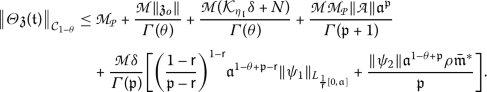By our assumptions we haveDividing both sides by δ and taking the limits of both sides as $$\delta \to \infty$$, we have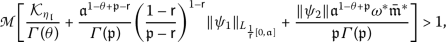which is a contradiction. Hence

$$\bigl\Vert {\varTheta }\mathfrak{z}(\mathfrak{t}) \bigr\Vert _{\mathcal{C}_{1-\theta }}< \delta .$$

This proves that Θ maps bounded sets to bounded sets.

Step 3. We prove that $${\varTheta }_{1}$$ is Lipschitz continuous. For $$\mathfrak{t}\in [0, \mathfrak{t}_{1}]$$, we have(3.4)

For $$\mathfrak{t}\in (\mathfrak{t}_{\mathfrak{l}}, \mathfrak{s}_{\mathfrak{l}}]$$, $$\mathfrak{l}=1, 2,\ldots, m$$, we have

$$\bigl\Vert {\varTheta }_{1}\mathfrak{z}_{1}( \mathfrak{t})- {\varTheta }_{1} \mathfrak{z}_{2}( \mathfrak{t}) \bigr\Vert _{\mathfrak{C}_{1-\theta }} \le \mathcal{K}_{\eta _{\mathfrak{l}}} \Vert \mathfrak{z}_{1}-\mathfrak{z}_{2} \Vert _{ \mathcal{C}_{1-\theta }}.$$
(3.5)

For $$\mathfrak{t}\in (\mathfrak{s}_{\mathfrak{l}}, \mathfrak{t}_{\mathfrak{l}+1}]$$, we have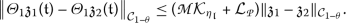(3.6)

Combining Eqs. (3.4)–(3.6), we getThis proves that $${\varTheta }_{1}$$ is a Lipschitz function with Lipschitz constant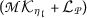.

Step 4. Let $$\{\mathfrak{z}_{n}(\mathfrak{t})\}$$ be a sequence in $$\varOmega _{\delta }$$ such that

$$\bigl\Vert \mathfrak{z}_{\mathfrak{n}}(\mathfrak{t})-\mathfrak{z}(t) \bigr\Vert \longrightarrow 0 \quad \text{as } \mathfrak{n}\longrightarrow \infty .$$

Sinceandare continuous functions with respect to the second and third variables, it follows thatBy assumptions (H1) and (H3) we haveSince $$\psi _{1}\in L_{\frac{1}{\mathsf{r}}[0, \mathfrak{a}]}$$ and $$\psi _{2}(\mathfrak{t})$$ is continuous, both functions on the right-hand side are integrable.

For all $$\mathfrak{t}\in \mathcal {J}$$, $$\mathfrak{z}_{n}, \mathfrak{z} \in \varOmega _{\mathfrak{\delta }}$$, considerBy the Lebesgue dominated convergence theorem

\begin{aligned} & \bigl\Vert {\varTheta }_{2} \mathfrak{z}_{n}(\mathfrak{t})-{\varTheta }_{2} \mathfrak{z}(\mathfrak{t}) \bigr\Vert _{\mathcal{C}_{1-\theta }} \longrightarrow 0 \quad \text{as } n \longrightarrow \infty . \end{aligned}

This proves the continuity of the operator $${\varTheta }_{2}$$.

Step 5. Let us prove that $${\varTheta }_{2}$$ is equicontinuous. For any $$\mathfrak{z} \in \varOmega _{\delta }$$ and $$\mathfrak{s}_{\mathfrak{l}}< \mathfrak{t}_{1}< \mathfrak{t}_{2}< \mathfrak{t}_{\mathfrak{l}+1}$$, $$\mathfrak{l}=0, 1, 2,\ldots, m$$, we have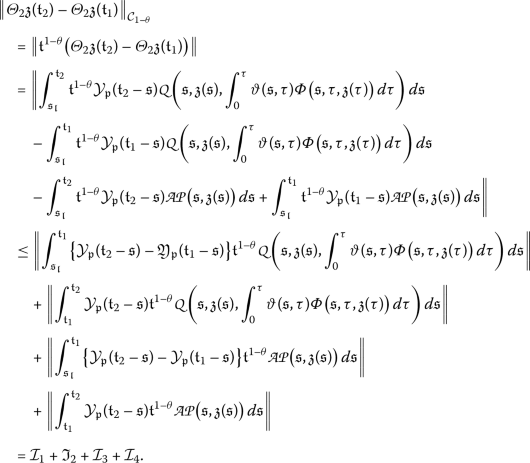By substituting $$(\mathfrak{t}_{1}-\mathfrak{s})=\mathfrak{s}_{1}$$ into $$\mathcal{I}_{1}$$ and $$\mathcal{I}_{3}$$ we haveBy the equicontinuity of $$(\mathfrak{p}-\mathfrak{q})$$-resolvent operator and the Lebesgue dominated convergence theorem both the integrals $$\mathcal{I}_{1}$$, $$\mathcal{I}_{3} \longrightarrow 0$$ as $$\mathfrak{t}_{2}\longrightarrow \mathfrak{t}_{1}$$.

Clearly, $$\mathcal{I}_{2}$$, $$\mathcal{I}_{4}\longrightarrow 0$$ as $$\mathfrak{t}_{2}\longrightarrow \mathfrak{t}_{1}$$, from which it follows that

$$\bigl\Vert {\varTheta }_{2}\mathfrak{z}(\mathfrak{t}_{2})-{ \varTheta }_{2} \mathfrak{z}(\mathfrak{t}_{1}) \bigr\Vert _{\mathcal{C}_{1-\theta }} \longrightarrow 0 \quad \text{as } \mathfrak{t}_{2} \longrightarrow \mathfrak{t}_{1}.$$

This proves the equicontinuity of $${\varTheta }_{2}$$.

Step 6. Let us prove that Θ is a condensing operator. We have to show that for any bounded subset $$\mathcal{D}\subset \varOmega _{\delta }$$,

$$\zeta \bigl( {\varTheta }(\mathcal{D}) \bigr) < \zeta {(\mathcal{D})}.$$

Since $${\varTheta }_{2}$$ is a continuous map, for any bounded set $$\mathcal{D} \subset \varOmega _{\delta }$$, there exists a countable set $$\mathcal{D}_{o}= \lbrace \mathfrak{z}_{n} \rbrace \subset \mathcal{D}$$ such that $$\zeta ( {\varTheta }_{2}(\mathcal{D}) )_{\mathcal{PC}_{1- \theta }} = \zeta ( {\varTheta }_{2}(\mathcal{D}_{o}) )_{ \mathcal{PC}_{1-\theta }}$$.

Since $${\varTheta }_{2}$$ is a bounded and equicontinuous operator, it follows that(3.7)

Equation (3.6) implies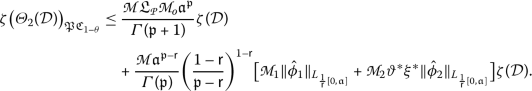Since $${\varTheta }_{1}$$ is a Lipschitz function with Lipschitz constant, for any bounded set $$\mathcal{D}\in \varOmega _{\delta }$$,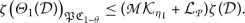(3.8)

As $${\varTheta }= {\varTheta }_{1}+{\varTheta }_{2}$$, we have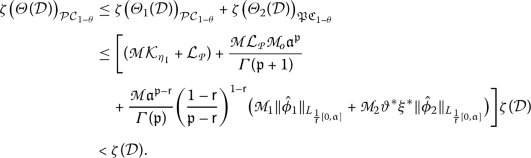Thus $${\varTheta }: \varOmega _{\delta }\longrightarrow \varOmega _{\delta }$$ is a condensing operator. Hence by Sadovskii’s fixed point theorem  operator Θ has at least one fixed point $$\mathfrak{z}\in \varOmega _{\delta }$$, which is a $$\mathcal{PC}_{1-\theta }$$ mild solution of the given system of equations. □

## Applications

Consider the following neutral Hilfer FDE with noninstantaneous impulsive condition on $$\mathcal {J}=[0, 1]$$: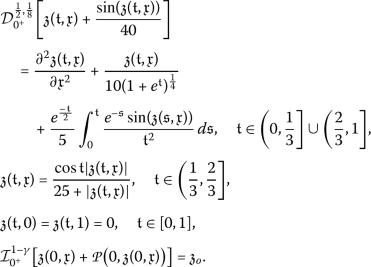(4.1)

Letandwith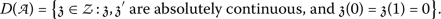The operatorgenerates an equicontinuous $$C_{o}$$-semigroup $$\mathcal{W}(\mathfrak{t})$$, ($$\mathfrak{t}\ge 0$$) onwith $$\|\mathcal{W}(\mathfrak{t})\|\le 1$$ for $$\mathfrak{t}\ge 0$$. On comparing with equation system (1.1), we havePut $$\mathfrak{z}(\mathfrak{t}, \mathfrak{x})= \mathfrak{z}(\mathfrak{t})$$. It is easy to see that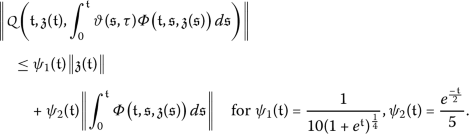Similarly,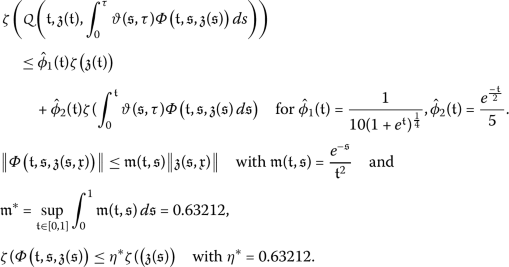In particular, take $$\mathsf{r}=\frac{1}{4}$$:

\begin{aligned} & \Vert \psi _{1} \Vert _{L_{\frac{1}{\mathsf{r}}[0,1]}}= \biggl( \int _{0}^{1} \biggl[\frac{1}{10 (1+e^{\mathfrak{t}})^{\frac{1}{4}}} \,d \mathfrak{t} \biggr]^{4} \biggr)^{\frac{1}{4}} =0.063 \quad \text{and} \\ & \Vert \psi _{2} \Vert _{L_{\frac{1}{\mathsf{r}}[0,1]}}= \biggl( \int _{0}^{1} \biggl[\frac{e^{\frac{-\mathfrak{t}}{2}}}{5} \,d \mathfrak{t} \biggr]^{4} \biggr)^{\frac{1}{4}} = 0.102. \end{aligned}

Bothand $$\eta _{\mathfrak{l}} ( \mathfrak{t}, \mathfrak{z}(\mathfrak{t}) )$$ are Lipschitz functions with Lipschitz constants $\mathcal{LP}=\frac{1}{40}$ and $$\mathcal{L}_{\eta _{\mathfrak{l}}}= \frac{1}{25}$$. Thus we have $$\mathfrak{p}= \frac{1}{2}$$, $$\mathfrak{q}= \frac{1}{8}$$, $$\theta = \frac{9}{16}$$, $$\mathsf{r}=\frac{1}{4}$$,, $$\vartheta ^{*}=1$$,,, $\mathcal{LP}=\frac{1}{40}$, $$\mathcal{L}_{\eta _{\mathfrak{l}}}= \frac{1}{25}$$, $$\|\psi _{1}\|=\|{ \hat{\phi }}_{1}\|= 0.063$$, $$\|\psi _{2}\|= \|{\hat{\phi }}_{2}\|=0.102$$, $$\eta ^{*}= \mathfrak{\bar{m}}^{*}=0.63212$$.

For these values, the first condition of Theorem 3.1 is satisfied:Similarly, we have the second condition: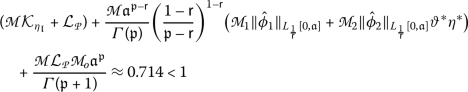Thus both conditions of Theorem 3.1 are satisfied. So the equation system (4.1) has at least one mild solution in $$\mathcal{PC}_{1-\theta }(\mathcal {J})$$.

## Conclusion

We established the existence of mild solutions for neutral fractional-order system involving the Hilfer fractional derivative in a Banach space by converting it into an integral form and hence applying Sadovskii’s fixed point technique. For future research work, for the proposed problem, we suggest stability analysis, multiple solutions, and singular solutions.

## References

1. Abdeljawad, T., Al-Mdallal, Q.M., Jarad, F.: Fractional logistic models in the frame of fractional operators generated by conformable derivatives. Chaos Solitons Fractals 119, 94–101 (2019). https://doi.org/10.1016/j.chaos.2018.12.015

2. Atangana, A., Gómez-Aguilar, J.F.: Numerical approximation of Riemann–Liouville definition of fractional derivative: from Riemann–Liouville to Atangana–Baleanu. Numer. Methods Partial Differ. Equ. 34(5), 1502–1523 (2018). https://doi.org/10.1002/num.22195

3. Banas, J.: Measures of noncompactness in the study of solutions of nonlinear differential and integral equations. Open Math. 10, 2003–2011 (2012). https://doi.org/10.2478/s11533-012-0120-9

4. Belmor, S., Ravichandran, C., Jarad, F.: Nonlinear generalized fractional differential equations with generalized fractional integral conditions. J. Taibah Univ. Sci. 14(1), 114–123 (2020). https://doi.org/10.1080/16583655.2019.1709265

5. Chalishajar, D., Ravichandran, C., Dhanalakshmi, S., Murugesu, R.: Existence of fractional impulsive functional integro-differential equations in Banach spaces. Appl. Syst. Innov. 2(2), 1–17 (2019). https://doi.org/10.3390/asi2020018

6. Chen, C., Li, M.: On fractional resolvent operator functions. Semigroup Forum 80, 121–142 (2010). https://doi.org/10.1007/s00233-009-9184-7

7. Chen, P., Zhang, X., Li, Y.: Existence of mild solutions of partial differential equations with non-instantaneous impulses. Electron. J. Differ. Equ. 2016, 241 (2016), 1–11, http://ejde.math.txstate.edu or http://ejde.math.unt.edu

8. Daher, S.J.: On a fixed point principle of Sadovskii. Nonlinear Anal., Theory Methods Appl. 2, 643–645 (1978). https://doi.org/10.1016/0362-546X(78)90012-3

9. Fu, X., Huang, R.: Existence of solutions for neutral integro-differential equations with state-dependent delay. Appl. Math. Comput. 224, 743–759 (2013). https://doi.org/10.1016/j.amc.2013.09.010

10. Furati, K.M., Kassim, M.D.: Existence and uniqueness for a problem involving Hilfer fractional derivative. Comput. Math. Appl. 64, 1616–1626 (2012). https://doi.org/10.1016/j.camwa.2012.01.009

11. Gambo, Y.Y., Ameen, R., Jarad, F., Abdeljawad, T.: Existence and uniqueness of solutions to fractional differential equations in the frame of generalized Caputo fractional derivatives. Adv. Differ. Equ. 2018, 134, 1–13 (2018). https://doi.org/10.1186/s13662-018-1594-y

12. Gou, H., Li, B.: Existence of mild solutions for Sobolev-type Hilfer fractional evolution equations with boundary conditions. Bound. Value Probl. 2018, 48 (2018). https://doi.org/10.1186/s13661-018-0965-3

13. Gu, H., Trujillo, J.J.: Existence of mild solution for evolution equations with Hilfer fractional derivative. Appl. Math. Comput. 257, 344–354 (2015). https://doi.org/10.1016/j.amc.2014.10.083

14. Hernández, E., ÓRegan, D.: On a new class of abstract impulsive differential equations. Proc. Am. Math. Soc. 141, 1641–1649 (2013). https://doi.org/10.1090/S0002-9939-2012-11613-2

15. Hilfer, R.: Fractional time evolution. In: Applications of Fractional Calculus in Physics, pp. 87–130 (2000). https://doi.org/10.1142/9789812817747_0002

16. Hilfer, R.: Applications of Fractional Calculus in Physics. World Scientific, Singapore (2000)

17. Jarad, F., Harikrishnan, S., Shah, K., Kanagarajan, K.: Existence and stability results to a class of fractional random implicit differential equations involving a generalized Hilfer fractional derivative. Discrete Contin. Dyn. Syst., Ser. S 13(3), 723–739 (2020). https://doi.org/10.3934/dcdss.2020040

18. Kilbas, A.A.A., Srivastava, H.M., Trujillo, J.J.: Theory and Applications of Fractional Differential Equations, vol. 204. Elsevier, Amsterdam (2006)

19. Lakshmikantham, V., Simeonov, P.S.: Theory of Impulsive Differential Equations. World Scientific Series on Modern Applied Mathematics, vol. 6. World Scientific, Singapore (1989). https://doi.org/10.1142/0906

20. Mophou, G.M.: Existence and uniqueness of mild solutions to impulsive fractional differential equations. Nonlinear Anal., Theory Methods Appl. 72, 1604–1615 (2010). https://doi.org/10.1016/j.na.2009.08.046

21. Morales-Delgado, V.F., Gómez-Aguilar, J.F., Escobar-Jimenez, R.F.: Fractional conformable attractors with low fractality. Math. Methods Appl. Sci. 41(16), 6378–6400 (2018). https://doi.org/10.1002/mma.5146

22. Morales-Delgado, V.F., Gómez-Aguilar, J.F., Escobar-Jiménez, R.F., Taneco-Hernández, M.A.: Fractional conformable derivatives of Liouville Caputo type with low-fractionality. Phys. A, Stat. Mech. Appl. 503, 424–438 (2018). https://doi.org/10.1016/j.physa.2018.03.018

23. Mursaleen, M., Noman, A.K.: The Hausdorff measure of noncompactness of matrix operators on some BK spaces. Oper. Matrices 5, 473–486 (2011)

24. Panda, S.K., Abdeljawad, T., Ravichandran, C.: Novel fixed point approach to Atangana–Baleanu fractional and $$L_{p}$$-Fredholm integral equations. Alex. Eng. J., 1–12 (2020, in press). https://doi.org/10.1016/j.aej.2019.12.027

25. Pandey, D.N., Das, S., Sukavanam, N.: Existence of solution for a second-order neutral differential equation with state dependent delay and non-instantaneous impulses. Int. J. Nonlinear Sci. 18, 145–155 (2014)

26. Perez, J.E.S., Gómez-Aguilar, J.F., Baleanu, D., Tchier, F.: Chaotic attractors with fractional conformable derivatives in the Liouville–Caputo sense and its dynamical behaviors. Entropy 20(5), 384 (2018). https://doi.org/10.3390/e20050384

27. Pierri, M., ÓRegan, D., Rolnik, V.: Existence of solutions for semi-linear abstract differential equations with non-instantaneous impulses. Appl. Math. Comput. 219, 6743–6749 (2013). https://doi.org/10.1090/S0002-9939-2012-11613-2

28. Podlubny, I.: Fractional Differential Equations. Academic Press, San Diego (1998). https://doi.org/10.2307/2653160

29. Ravichandran, C., Logeswari, K., Jarad, F.: New results on existence in the framework of Atangana–Baleanu derivative for fractional integro-differential equations. Chaos Solitons Fractals 125, 194–200 (2019). https://doi.org/10.1016/j.chaos.2019.05.014

30. Shu, X.B., Lai, Y., Chen, Y.: The existence of mild solutions for impulsive fractional partial differential equations. Nonlinear Anal., Theory Methods Appl. 74, 2003–2011 (2011). https://doi.org/10.1016/j.na.2010.11.007

31. Sousa, J.: Existence of mild solutions to Hilfer fractional evolution equations in Banach space (2018). arXiv:1812.02213. arXiv preprint

32. Subashini, R., Jothimani, K., Saranya, S., Ravichandran, C.: On the results of Hilfer fractional derivative with nonlocal conditions. Int. J. Pure Appl. Math. 118(11), 277–289 (2018). https://doi.org/10.12732/ijpam.v118i11.33

33. Subashini, R., Ravichandran, C.: On the results of nonlocal Hilfer fractional semilinear differential inclusions. Proc. Jangjeon Math. Soc. 22(2), 249–267 (2019). https://doi.org/10.17777/pjms2019.22.2.249

34. Subashini, R., Ravichandran, C., Jothimani, K., Baskonus, H.M.: Existence results of Hilfer integro-differential equations with fractional order. Discrete Contin. Dyn. Syst., Ser. S 13(3), 911–923 (2020). https://doi.org/10.3934/dcdss.2020053

35. Suganya, S., Baleanu, D., Kalamani, P., Arjunan, M.M.: On fractional neutral integro-differential systems with state-dependent delay and non-instantaneous impulses. Adv. Differ. Equ. 2015, 372, 1–39 (2015). https://doi.org/10.1186/s13662-015-0709-y

36. Wang, J., Zhang, Y.: Nonlocal initial value problems for differential equations with Hilfer fractional derivative. Appl. Math. Comput. 266, 850–859 (2015). https://doi.org/10.1016/j.amc.2015.05.144

37. Yang, M., Wang, Q.: Existence of mild solutions for a class of Hilfer fractional evolution equations with nonlocal conditions. Fract. Calc. Appl. Anal. 20, 679–705 (2017). https://doi.org/10.1515/fca-2017-0036

38. Ye, R.: Existence of solutions for impulsive partial neutral functional differential equation with infinite delay. Nonlinear Anal., Theory Methods Appl. 73, 155–162 (2010). https://doi.org/10.1016/j.na.2010.03.008

39. Yépez-Martínez, H., Gómez-Aguilar, J.F.: Fractional sub-equation method for Hirota–Satsuma-coupled KdV equation and coupled mKdV equation using the Atanganás conformable derivative. Waves Random Complex Media 29(4), 678–693 (2019). https://doi.org/10.1080/17455030.2018.1464233

40. Zhou, Y., Jiao, F.: Existence of mild solutions for fractional neutral evolution equations. Comput. Math. Appl. 59, 1063–1077 (2010). https://doi.org/10.1016/j.camwa.2009.06.026

### Acknowledgements

The first author is highly thankful to the Council of Scientific and Industrial Research (CSIR)-New Delhi, India, for their financial support with grant no. 09/1051(0017)/2018-EMR-I. The third author (Thabet Abdeljawad) would like to thank Prince Sultan University for funding this work through research group in Applied Mathematics (NAMAM) group number RG-DES-201701-17.

Not applicable.

## Funding

Prince Sultan University for funding this work through research group in Applied Mathematics (NAMAM) group number RG-DES-201701-17.

## Author information

Authors

### Contributions

All authors have equal contributions in this paper. All authors read and approved the final manuscript.

### Corresponding author

Correspondence to Thabet Abdeljawad.

## Ethics declarations

### Competing interests

The authors have no competing interests regarding the publication of this paper.

## Rights and permissions

Open Access This article is licensed under a Creative Commons Attribution 4.0 International License, which permits use, sharing, adaptation, distribution and reproduction in any medium or format, as long as you give appropriate credit to the original author(s) and the source, provide a link to the Creative Commons licence, and indicate if changes were made. The images or other third party material in this article are included in the article’s Creative Commons licence, unless indicated otherwise in a credit line to the material. If material is not included in the article’s Creative Commons licence and your intended use is not permitted by statutory regulation or exceeds the permitted use, you will need to obtain permission directly from the copyright holder. To view a copy of this licence, visit http://creativecommons.org/licenses/by/4.0/.

Reprints and Permissions